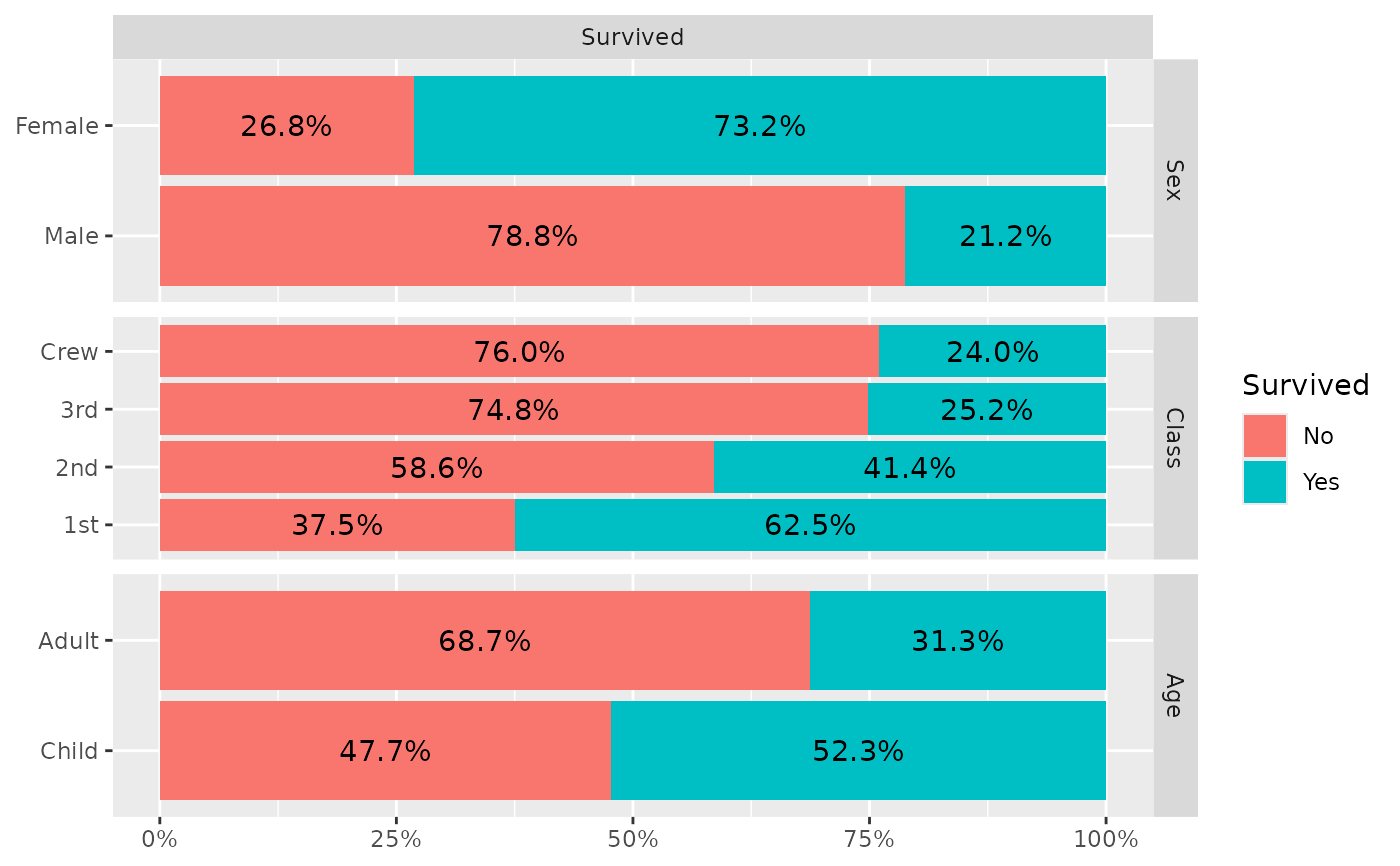Plot column or row percentage using bar plots.

ggally_colbar(
data,
mapping,
label_format = scales::label_percent(accuracy = 0.1),
...,
remove_background = FALSE,
remove_percentage_axis = FALSE,
reverse_fill_levels = FALSE,
geom_bar_args = NULL
)

ggally_rowbar(
data,
mapping,
label_format = scales::label_percent(accuracy = 0.1),
...,
remove_background = FALSE,
remove_percentage_axis = FALSE,
reverse_fill_levels = TRUE,
geom_bar_args = NULL
)

## Arguments

data data set using aesthetics being used formatter function for displaying proportions, not taken into account if a label aesthetic is provided in mapping other arguments passed to geom_text(...) should the panel.background be removed? should percentage axis be removed? Removes the y-axis for ggally_colbar() and x-axis for ggally_rowbar() should the levels of the fill variable be reversed? other arguments passed to geom_bar(...)

## Author

Joseph Larmarange

## Examples

# Small function to display plots only if it's interactive
p_ <- GGally::print_if_interactive

data(tips, package = "reshape")
p_(ggally_colbar(tips, mapping = aes(x = smoker, y = sex)))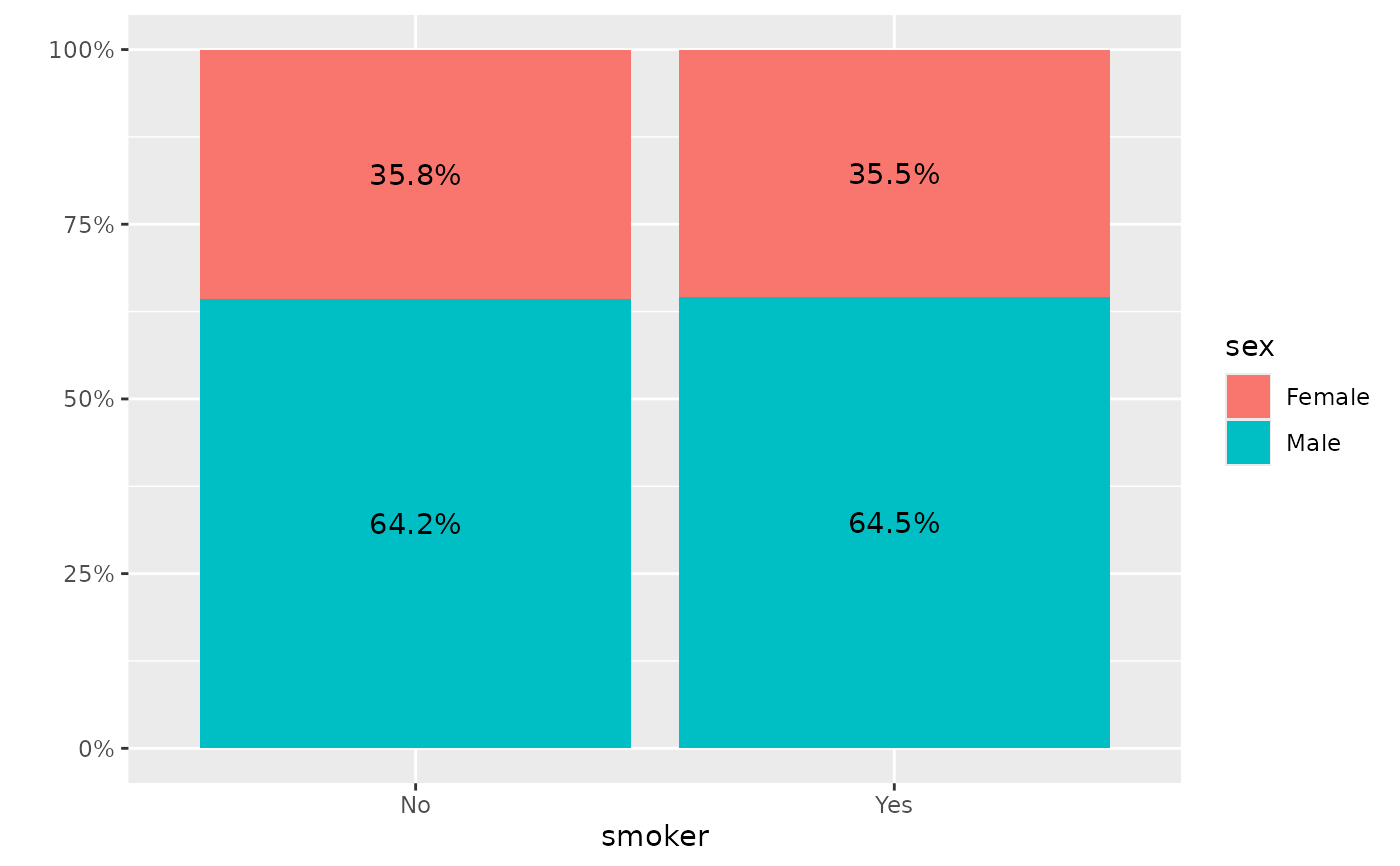p_(ggally_rowbar(tips, mapping = aes(x = smoker, y = sex)))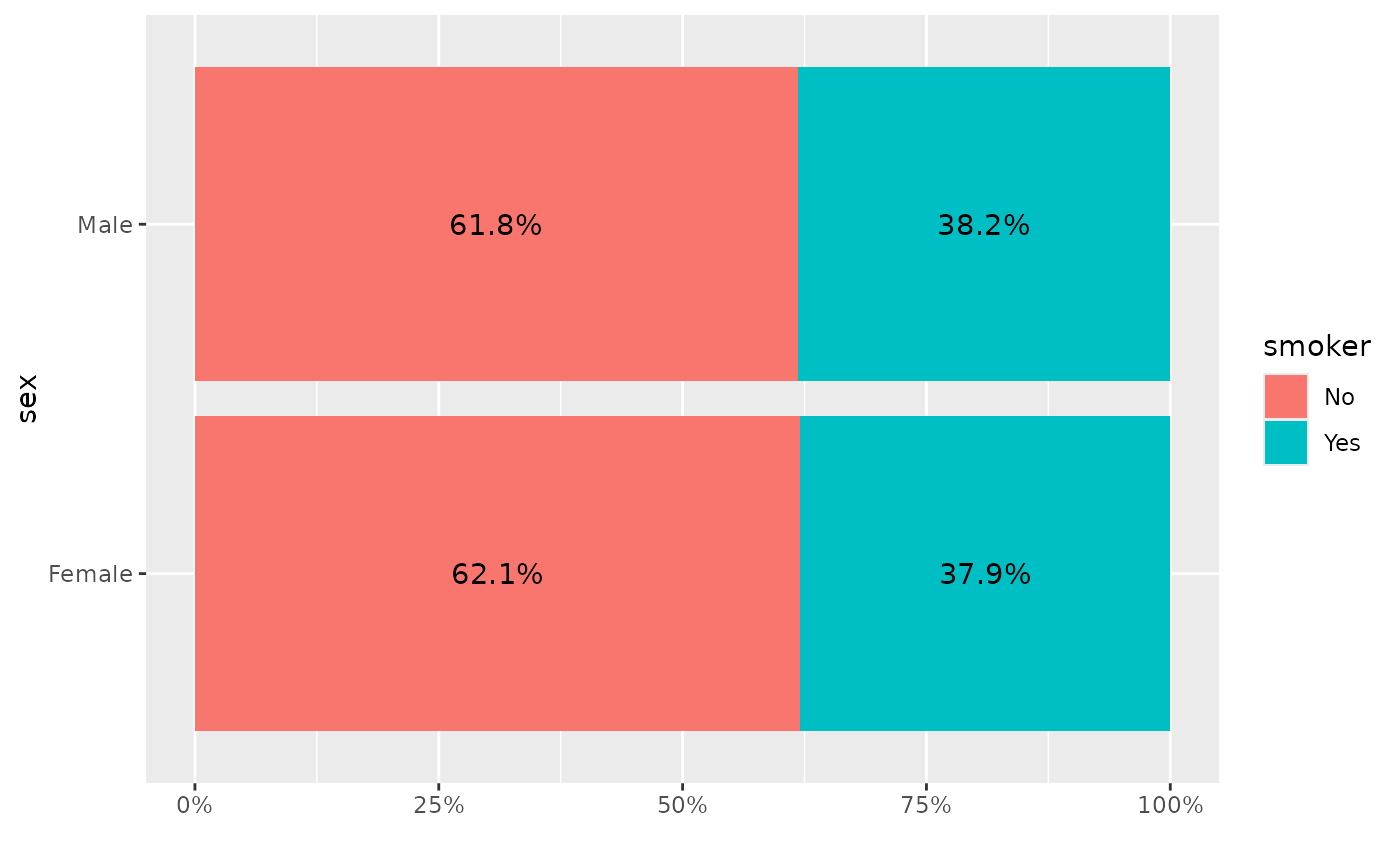# change labels' size
p_(ggally_colbar(tips, mapping = aes(x = smoker, y = sex), size = 8))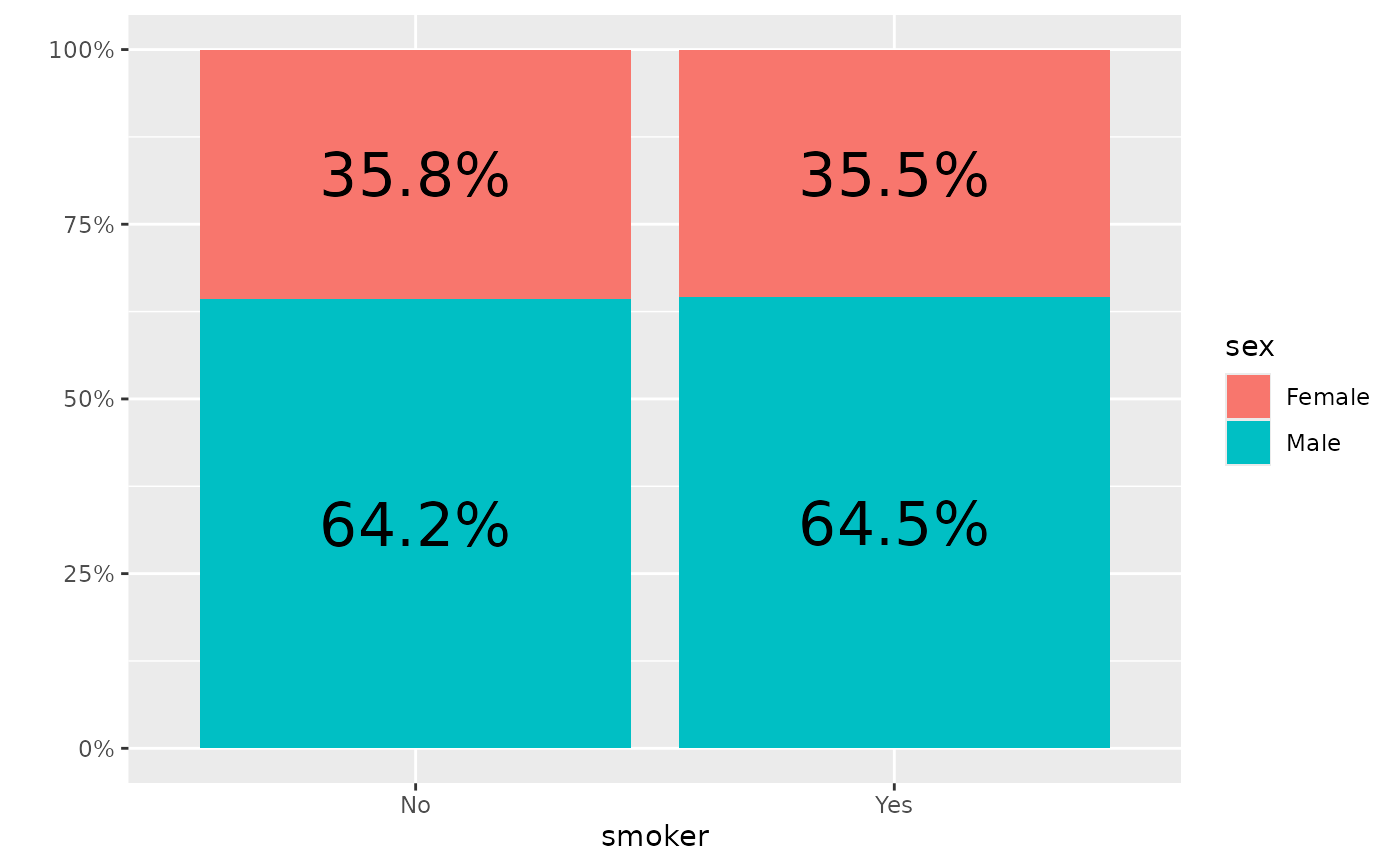# change labels' colour and use bold
p_(ggally_colbar(tips, mapping = aes(x = smoker, y = sex),
colour = "white", fontface = "bold"))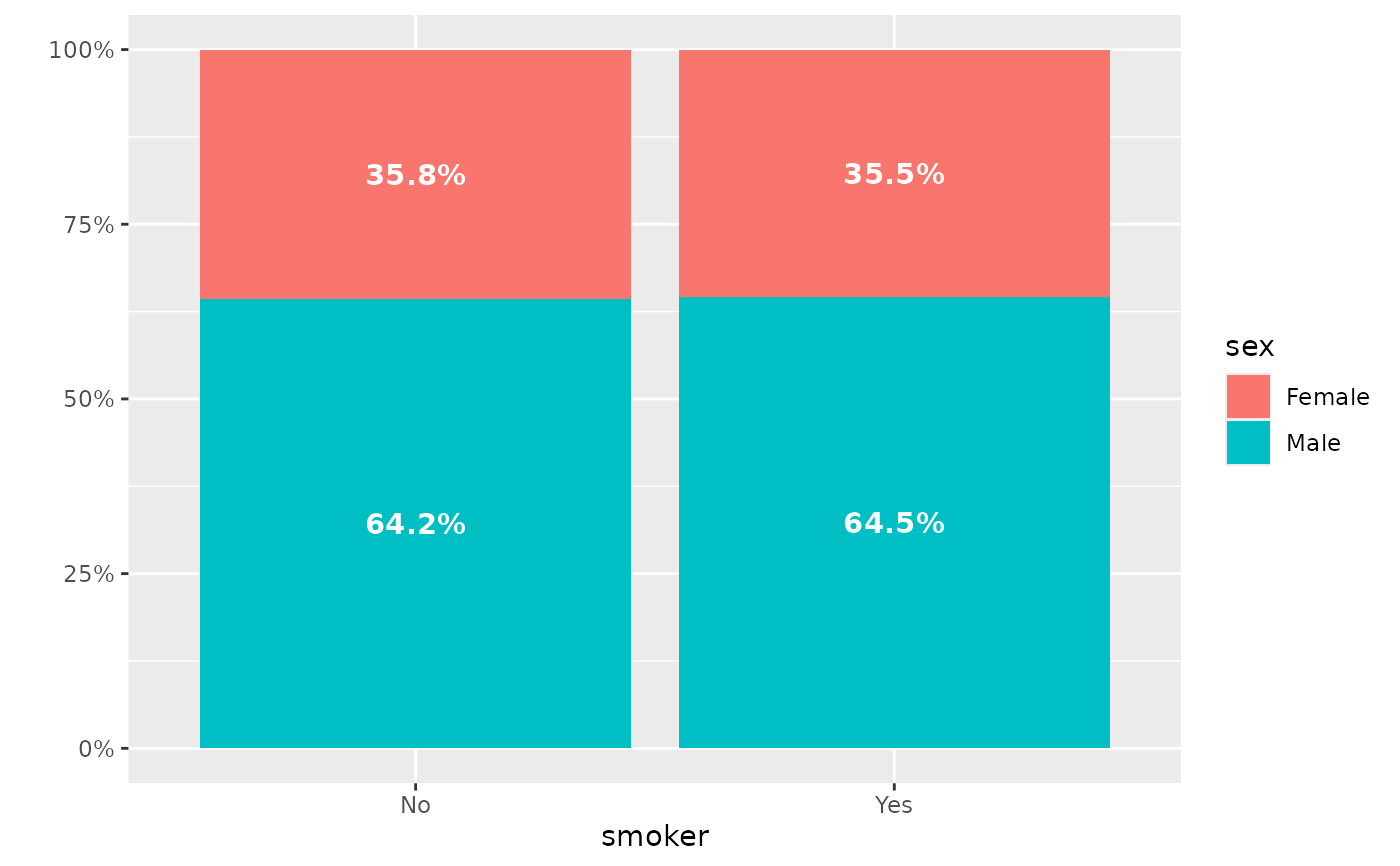# display number of observations instead of proportions
p_(ggally_colbar(tips, mapping = aes(x = smoker, y = sex, label = after_stat(count))))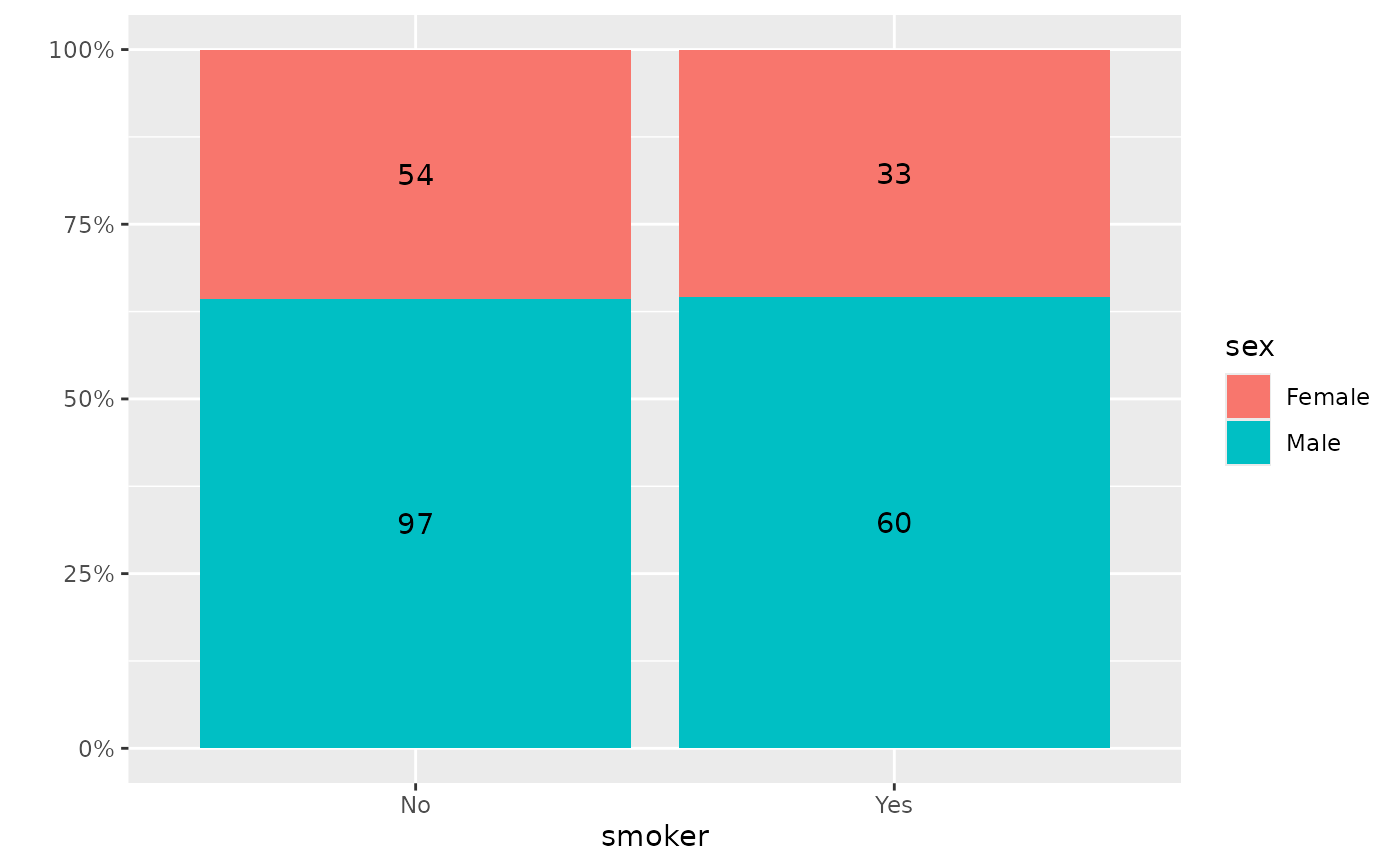# custom bar width
p_(ggally_colbar(tips, mapping = aes(x = smoker, y = sex), geom_bar_args = list(width = .5)))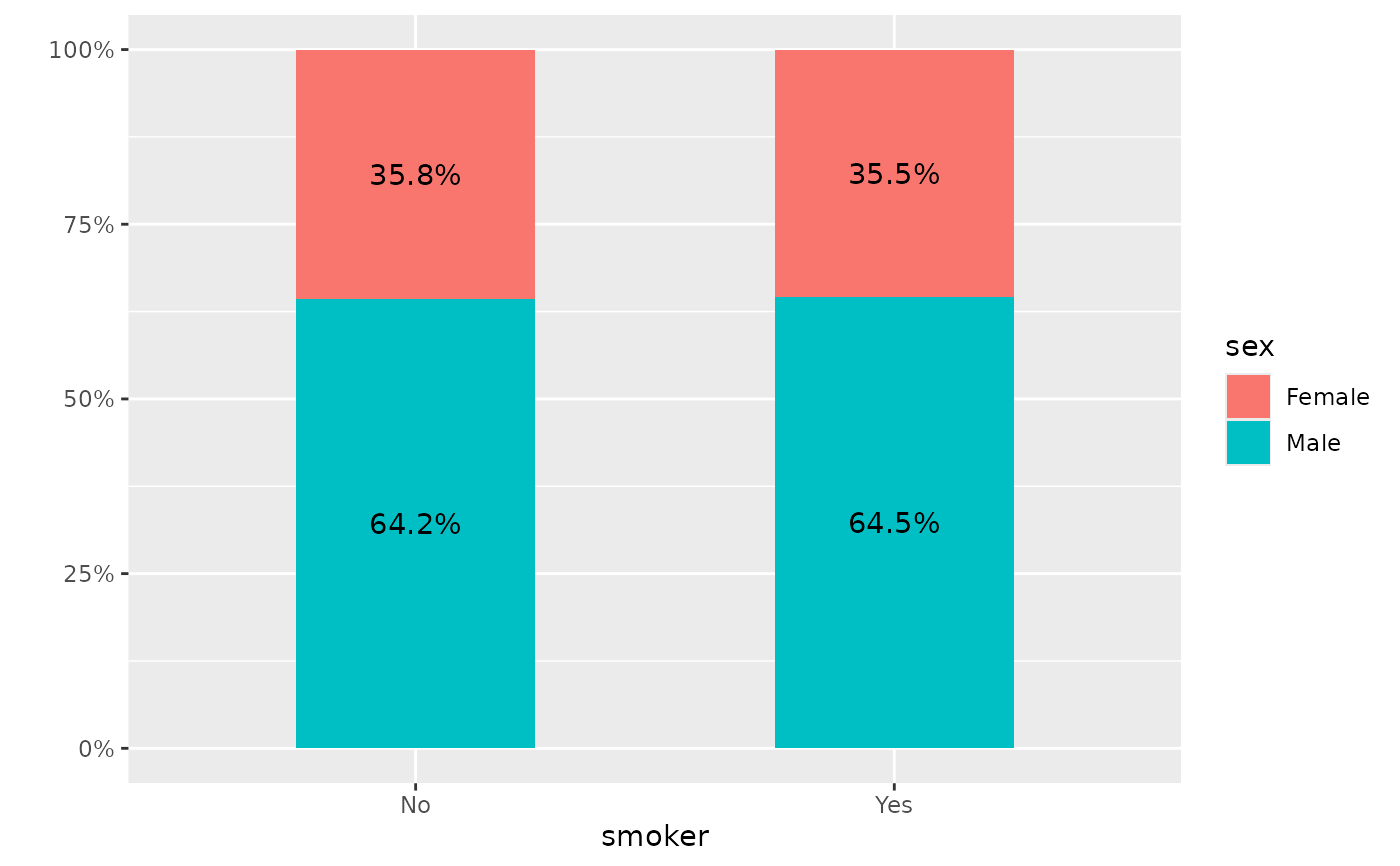# change format of labels
p_(ggally_colbar(tips, mapping = aes(x = smoker, y = sex),
label_format = scales::label_percent(accuracy = .01, decimal.mark = ",")))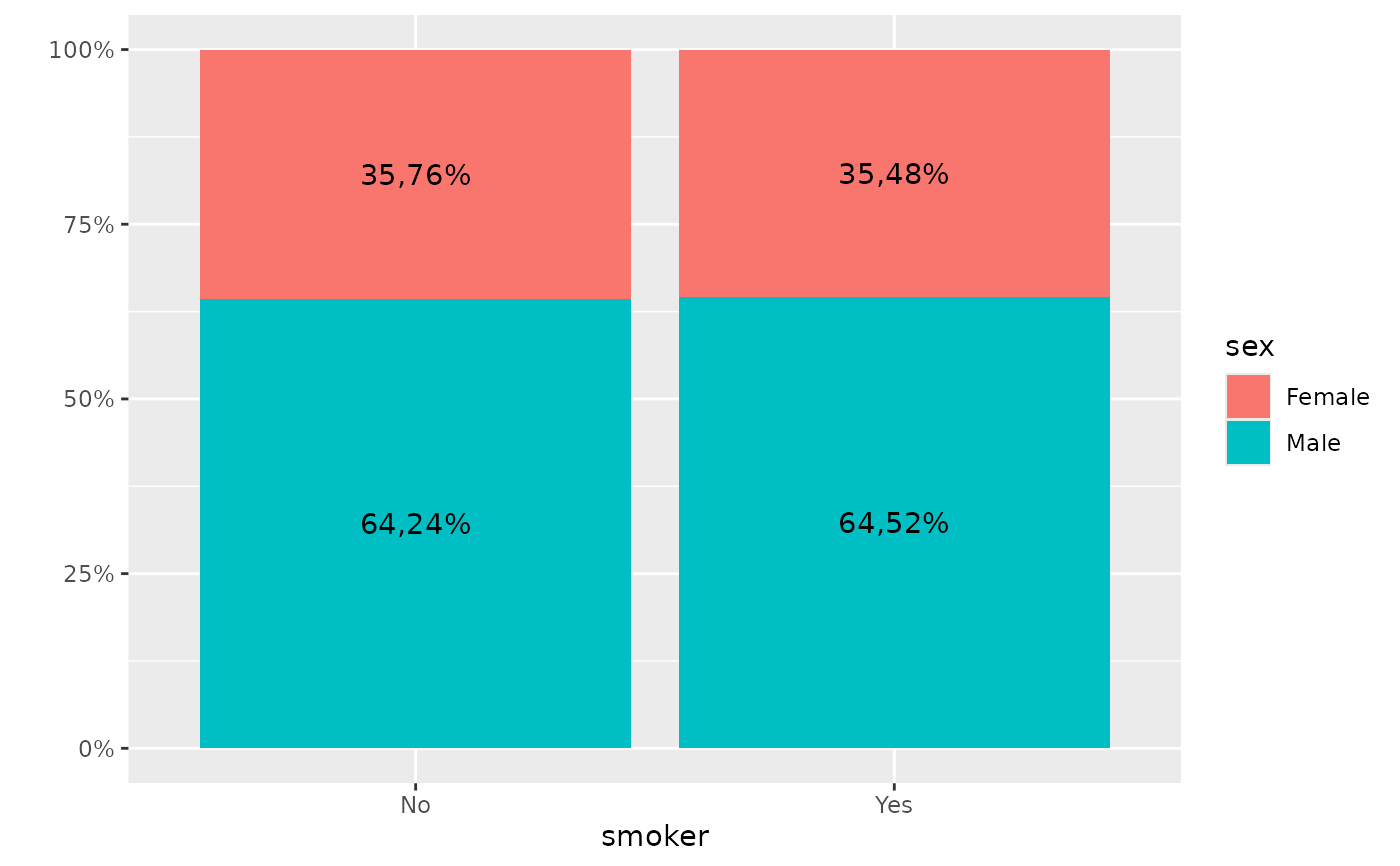p_(ggduo(
data = as.data.frame(Titanic),
mapping = aes(weight = Freq),
columnsX = "Survived",
columnsY = c("Sex", "Class", "Age"),
types = list(discrete = "rowbar"),
legend = 1
))